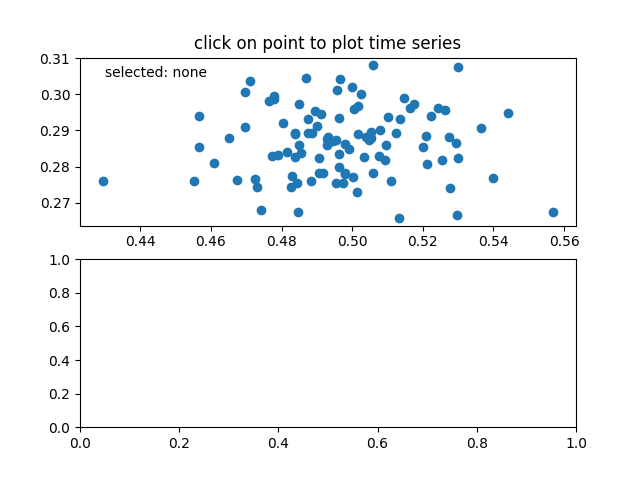# Data browser#

Connecting data between multiple canvases.

This example covers how to interact data with multiple canvases. This lets you select and highlight a point on one axis, and generating the data of that point on the other axis.

Note

This example exercises the interactive capabilities of Matplotlib, and this will not appear in the static documentation. Please run this code on your machine to see the interactivity.

You can copy and paste individual parts, or download the entire example using the link at the bottom of the page.import numpy as np

class PointBrowser:
"""
Click on a point to select and highlight it -- the data that
generated the point will be shown in the lower axes.  Use the 'n'
and 'p' keys to browse through the next and previous points
"""

def __init__(self):
self.lastind = 0

self.text = ax.text(0.05, 0.95, 'selected: none',
transform=ax.transAxes, va='top')
self.selected, = ax.plot([xs], [ys], 'o', ms=12, alpha=0.4,
color='yellow', visible=False)

def on_press(self, event):
if self.lastind is None:
return
if event.key not in ('n', 'p'):
return
if event.key == 'n':
inc = 1
else:
inc = -1

self.lastind += inc
self.lastind = np.clip(self.lastind, 0, len(xs) - 1)
self.update()

def on_pick(self, event):

if event.artist != line:
return True

N = len(event.ind)
if not N:
return True

# the click locations
x = event.mouseevent.xdata
y = event.mouseevent.ydata

distances = np.hypot(x - xs[event.ind], y - ys[event.ind])
indmin = distances.argmin()
dataind = event.ind[indmin]

self.lastind = dataind
self.update()

def update(self):
if self.lastind is None:
return

dataind = self.lastind

ax2.clear()
ax2.plot(X[dataind])

ax2.text(0.05, 0.9, f'mu={xs[dataind]:1.3f}\nsigma={ys[dataind]:1.3f}',
transform=ax2.transAxes, va='top')
ax2.set_ylim(-0.5, 1.5)
self.selected.set_visible(True)
self.selected.set_data(xs[dataind], ys[dataind])

self.text.set_text('selected: %d' % dataind)
fig.canvas.draw()

if __name__ == '__main__':
import matplotlib.pyplot as plt
# Fixing random state for reproducibility
np.random.seed(19680801)

X = np.random.rand(100, 200)
xs = np.mean(X, axis=1)
ys = np.std(X, axis=1)

fig, (ax, ax2) = plt.subplots(2, 1)
ax.set_title('click on point to plot time series')
line, = ax.plot(xs, ys, 'o', picker=True, pickradius=5)

browser = PointBrowser()

fig.canvas.mpl_connect('pick_event', browser.on_pick)
fig.canvas.mpl_connect('key_press_event', browser.on_press)

plt.show()


Gallery generated by Sphinx-Gallery